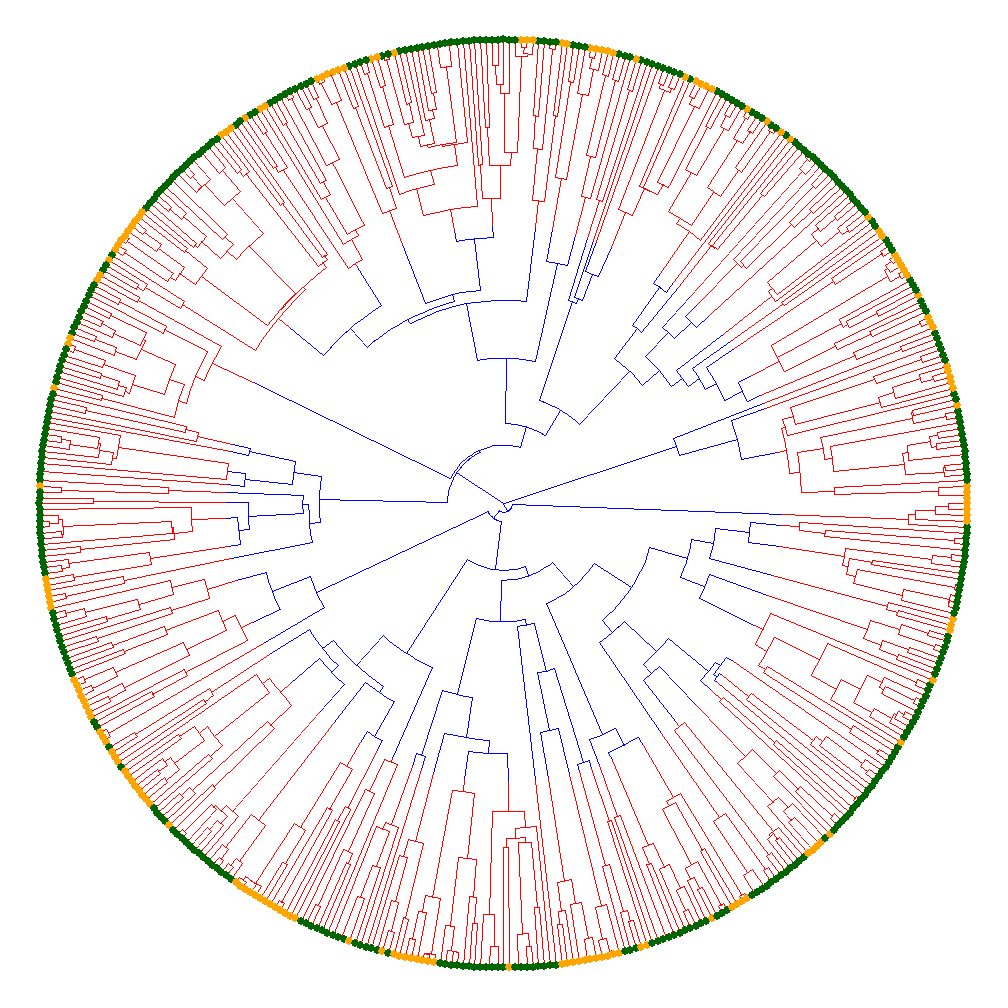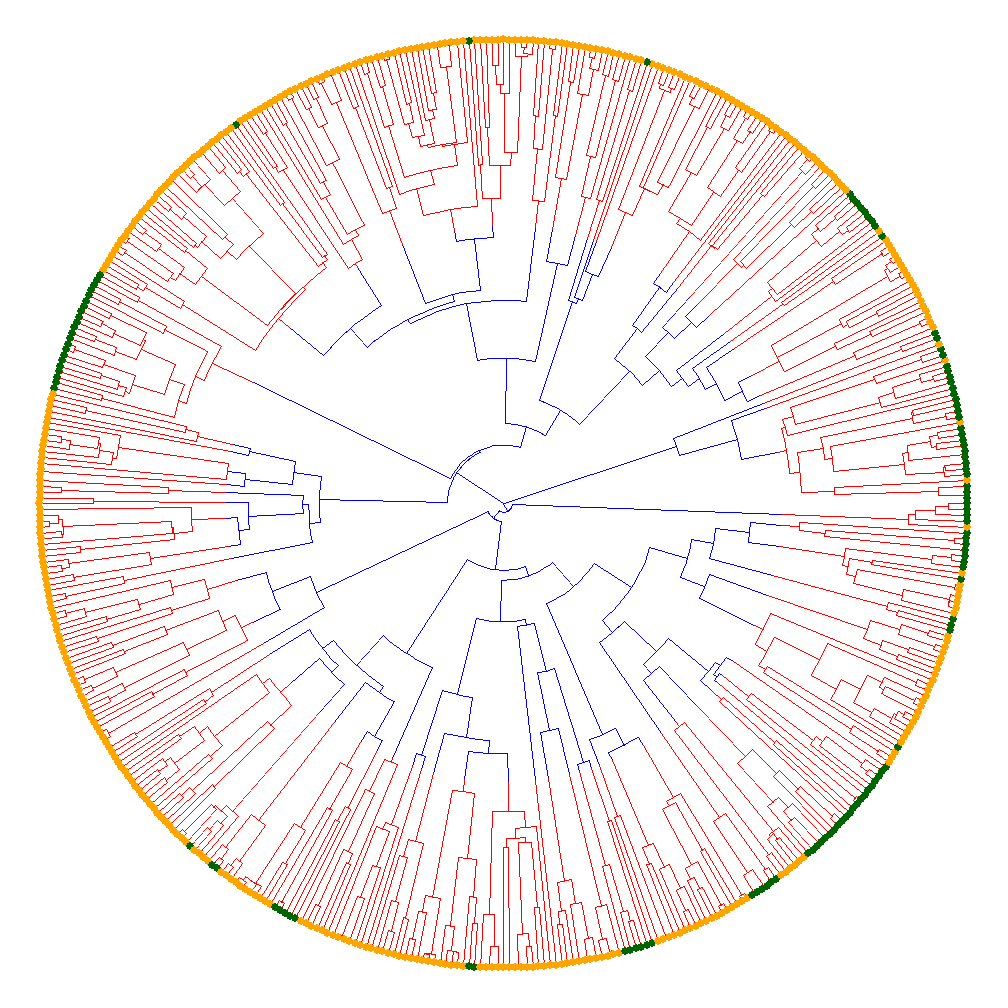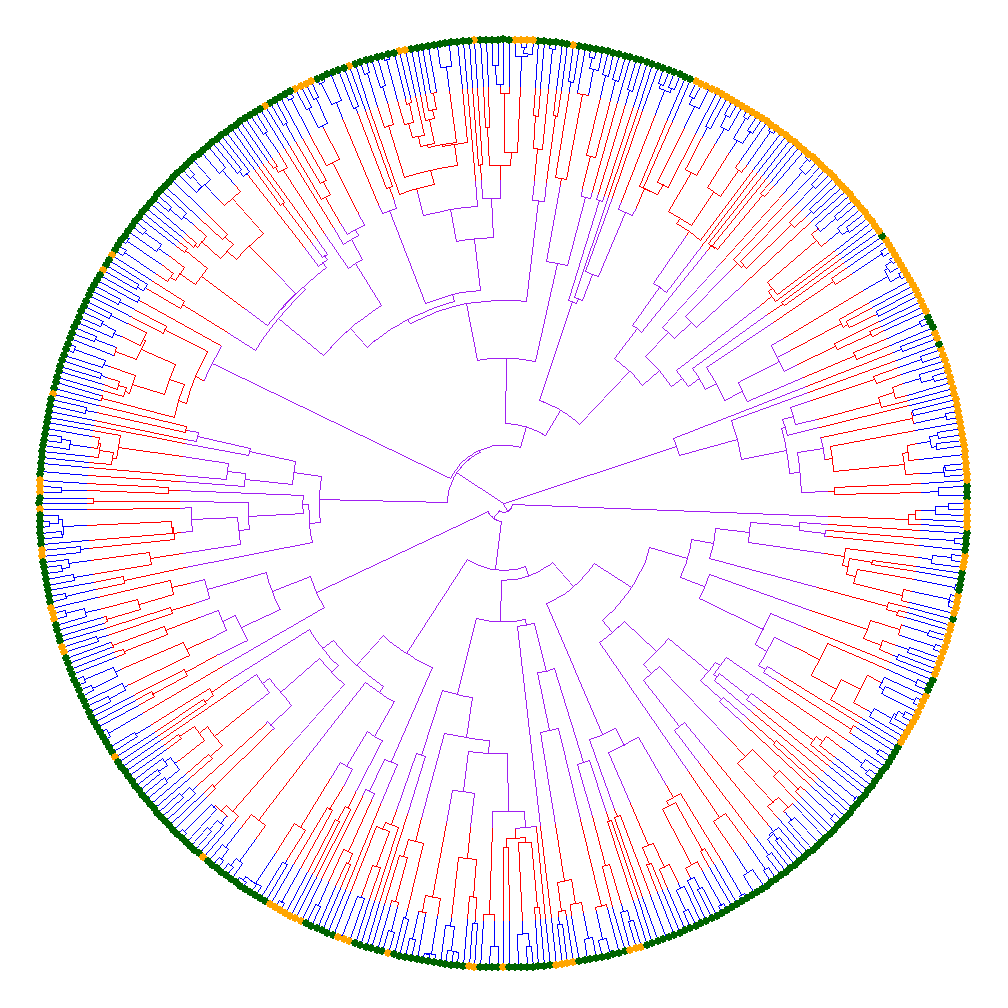## Friday, November 23, 2018

### Testing for differences in the rate (or process) of discrete character evolution between time periods

The phytools function `fitmultiMk` is designed to fit an 'extended' Mk model in which the parameters of the substitution process for the discrete character are allowed to change among different edges of the tree (and even within an edge) as specified by the user.

The model implemented in the function is relatively simple and it is closely related to other models that permit a heterogeneous rate of discrete character evolution on the tree. For instance, the model of Pagel (1994), usually interpreted as a 'correlational' model, is actually one in which the rate or process of evolution for one binary character is influenced by the state of a second (or vice versa). The 'covarion' model of Tuffley & Steel (1997) permits a discrete trait to evolve under a process in which it is affected by an observed hidden state that turns evolution of the character 'on' or 'off.' This idea was subsequently extended and generalized to phylogenetic comparative analyses by Beaulieu et al. (2013). `fitmultiMk` thus treats a very specific circumstance in which we have mapped onto the tree a priori a particular hypothesis for how we suppose that the rate or process of discrete character evolution varies on the tree, thus closely a kin to the model of O'Meara et al.
(2006), but for discrete characters. (One of my collaborators also pointed out that `fitmultiMk` is also closely related to a test for dN/dS ratio variation among pre-specified branches of the tree called the 'codon branch model' and originally published by Yang 1998.)

One intriguing attribute of this model is that it allows us to fit a model in which the rate or process of evolution differs among different time periods on the tree - not merely among clades and edges. This is also true in O'Meara et al. (2006), but is unlike (for instance) a Pagel (1994) type approach. I was suspicious about how well this would work because I imagined that the relatively small number of edges towards the root of the tree wouldn't allow to fit a separate rate to that time period. At a certain point, this must be true - but it is also not difficult to find conditions in which `fitmultiMk` applied to time-periods rather than edges or clades actually works pretty well.

Here I'll give some examples. First, I'm going to simulate and then fit a couple of two-rate (that is, two-era) models: one in which the rate is initially low & then increased; followed by a second in which the rate of evolution is initially low, but then increased. After that, I'll simulate & fit a three-era model.

Here are the two rate/era models:

``````library(phytools)
``````
``````## Loading required package: ape
``````
``````## Loading required package: maps
``````
``````library(lmtest)
``````
``````## Loading required package: zoo
``````
``````##
## Attaching package: 'zoo'
``````
``````## The following objects are masked from 'package:base':
##
##     as.Date, as.Date.numeric
``````
``````tree<-pbtree(n=500,scale=100)
tt<-make.era.map(tree,c(0,60))
## slow then fast:
Q<-setNames(list(
matrix(0.002*c(-1,1,1,-1),2,2,
dimnames=list(letters[1:2],letters[1:2])),
matrix(0.01*c(-1,1,1,-1),2,2,
dimnames=list(letters[1:2],letters[1:2]))),
1:2)
Q
``````
``````## \$`1`
##        a      b
## a -0.002  0.002
## b  0.002 -0.002
##
## \$`2`
##       a     b
## a -0.01  0.01
## b  0.01 -0.01
``````
``````x<-sim.multiMk(tt,Q)
cols<-setNames(c("blue","red"),1:2)
plot(tt,cols,ftype="off",lwd=1,type="fan")
par(fg="transparent")
tiplabels(pie=to.matrix(x,levels(x)),cex=0.2,
piecol=c("darkgreen","orange"))
````````````par(fg="black")
fit.single<-fitMk(tree,x)
fit.single
``````
``````## Object of class "fitMk".
##
## Fitted (or set) value of Q:
##           a         b
## a -0.008851  0.008851
## b  0.008851 -0.008851
##
## Fitted (or set) value of pi:
##   a   b
## 0.5 0.5
##
## Log-likelihood: -209.45609
##
## Optimization method used was "nlminb"
``````
``````fit.multi<-fitmultiMk(tt,x,opt.method="optim")
fit.multi
``````
``````## Object of class "fitmultiMk".
##
## Fitted value of Q:
##           a         b
## a -0.001487  0.001487
## b  0.001487 -0.001487
##
## Fitted value of Q:
##           a         b
## a -0.009942  0.009942
## b  0.009942 -0.009942
##
## Fitted (or set) value of pi:
##   a   b
## 0.5 0.5
##
## Log-likelihood: -207.477428
##
## Optimization method used was "optim"
``````
``````lrtest(fit.single,fit.multi)
``````
``````## Warning in modelUpdate(objects[[i - 1]], objects[[i]]): original model was
## of class "fitMk", updated model is of class "fitmultiMk"
``````
``````## Likelihood ratio test
##
## Model 1: fit.single
## Model 2: fit.multi
##   #Df  LogLik Df  Chisq Pr(>Chisq)
## 1   1 -209.46
## 2   2 -207.48  1 3.9573    0.04667 *
## ---
## Signif. codes:  0 '***' 0.001 '**' 0.01 '*' 0.05 '.' 0.1 ' ' 1
``````
``````## fast then slow:
Q<-setNames(list(
matrix(0.01*c(-1,1,1,-1),2,2,
dimnames=list(letters[1:2],letters[1:2])),
matrix(0.002*c(-1,1,1,-1),2,2,
dimnames=list(letters[1:2],letters[1:2]))),
1:2)
Q
``````
``````## \$`1`
##       a     b
## a -0.01  0.01
## b  0.01 -0.01
##
## \$`2`
##        a      b
## a -0.002  0.002
## b  0.002 -0.002
``````
``````x<-sim.multiMk(tt,Q)
cols<-setNames(c("blue","red"),1:2)
plot(tt,cols,ftype="off",lwd=1,type="fan")
par(fg="transparent")
tiplabels(pie=to.matrix(x,levels(x)),cex=0.2,
piecol=c("darkgreen","orange"))
````````````par(fg="black")
fit.single<-fitMk(tree,x)
fit.single
``````
``````## Object of class "fitMk".
##
## Fitted (or set) value of Q:
##           a         b
## a -0.002806  0.002806
## b  0.002806 -0.002806
##
## Fitted (or set) value of pi:
##   a   b
## 0.5 0.5
##
## Log-likelihood: -99.586308
##
## Optimization method used was "nlminb"
``````
``````fit.multi<-fitmultiMk(tt,x,opt.method="optim")
fit.multi
``````
``````## Object of class "fitmultiMk".
##
## Fitted value of Q:
##           a         b
## a -0.008296  0.008296
## b  0.008296 -0.008296
##
## Fitted value of Q:
##          a        b
## a -0.00214  0.00214
## b  0.00214 -0.00214
##
## Fitted (or set) value of pi:
##   a   b
## 0.5 0.5
##
## Log-likelihood: -96.381071
##
## Optimization method used was "optim"
``````
``````lrtest(fit.single,fit.multi)
``````
``````## Warning in modelUpdate(objects[[i - 1]], objects[[i]]): original model was
## of class "fitMk", updated model is of class "fitmultiMk"
``````
``````## Likelihood ratio test
##
## Model 1: fit.single
## Model 2: fit.multi
##   #Df  LogLik Df  Chisq Pr(>Chisq)
## 1   1 -99.586
## 2   2 -96.381  1 6.4105    0.01134 *
## ---
## Signif. codes:  0 '***' 0.001 '**' 0.01 '*' 0.05 '.' 0.1 ' ' 1
``````

Although not the default optimization method, I found that `opt.method="optim"` seemed to work a little better than `opt.method="nlminb"` (the default) for whatever reason.

I would generally expect the high-rate / low-rate model to get closer to the true generating parameters because there should be more changes on the relatively shorter total internal branch lengths if the rate is higher. That intuition seems to correspond to the results we found above.

We can also try three different eras & see how that works:

``````tt<-make.era.map(tree,c(0,70,90))
Q<-setNames(list(
matrix(0.005*c(-1,1,1,-1),2,2,dimnames=list(letters[1:2],letters[1:2])),
matrix(0.01*c(-1,1,1,-1),2,2,dimnames=list(letters[1:2],letters[1:2])),
matrix(0.001*c(-1,1,1,-1),2,2,dimnames=list(letters[1:2],letters[1:2]))),
1:3)
Q
``````
``````## \$`1`
##        a      b
## a -0.005  0.005
## b  0.005 -0.005
##
## \$`2`
##       a     b
## a -0.01  0.01
## b  0.01 -0.01
##
## \$`3`
##        a      b
## a -0.001  0.001
## b  0.001 -0.001
``````
``````x<-sim.multiMk(tt,Q)
cols<-setNames(c("purple","red","blue"),1:3)
plot(tt,cols,ftype="off",lwd=1,type="fan")
par(fg="transparent")
tiplabels(pie=to.matrix(x,levels(x)),cex=0.2,
piecol=c("darkgreen","orange"))
````````````par(fg="black")
fit.single<-fitMk(tree,x)
fit.single
``````
``````## Object of class "fitMk".
##
## Fitted (or set) value of Q:
##           a         b
## a -0.005102  0.005102
## b  0.005102 -0.005102
##
## Fitted (or set) value of pi:
##   a   b
## 0.5 0.5
##
## Log-likelihood: -149.374324
##
## Optimization method used was "nlminb"
``````
``````fit.multi<-fitmultiMk(tt,x,opt.method="optim")
fit.multi
``````
``````## Object of class "fitmultiMk".
##
## Fitted value of Q:
##          a        b
## a -0.00303  0.00303
## b  0.00303 -0.00303
##
## Fitted value of Q:
##           a         b
## a -0.009751  0.009751
## b  0.009751 -0.009751
##
## Fitted value of Q:
##           a         b
## a -0.002294  0.002294
## b  0.002294 -0.002294
##
## Fitted (or set) value of pi:
##   a   b
## 0.5 0.5
##
## Log-likelihood: -144.906686
##
## Optimization method used was "optim"
``````
``````lrtest(fit.single,fit.multi)
``````
``````## Warning in modelUpdate(objects[[i - 1]], objects[[i]]): original model was
## of class "fitMk", updated model is of class "fitmultiMk"
``````
``````## Likelihood ratio test
##
## Model 1: fit.single
## Model 2: fit.multi
##   #Df  LogLik Df  Chisq Pr(>Chisq)
## 1   1 -149.37
## 2   3 -144.91  2 8.9353    0.01147 *
## ---
## Signif. codes:  0 '***' 0.001 '**' 0.01 '*' 0.05 '.' 0.1 ' ' 1
``````

1.Does the function allow you to actually identify the location/timing of shifts between regimes? Or is it just the number of regimes? Thanks. Neil

1.Neil. This function is not designed to identify rate shifts, but to test a hypothesis that we propose that there is a (or one or more) rate shift(s) on the tree. I have a function to the equivalent of what I think you have in mind - but for continuous characters. It is called rateshift. I could try to extend it to discrete characters & may. I don't think it's a bad idea.

- Liam

2.thanks a lot. N

2.This comment has been removed by the author.

Note: due to the very large amount of spam, all comments are now automatically submitted for moderation.